# Arrow impossibility theorem

(diff) ← Older revision | Latest revision (diff) | Newer revision → (diff)

In 1951, K. Arrow [a1] discovered a troubling result about decisions involving three or more alternatives. After posing innocuous conditions that seemingly are satisfied by all reasonable procedures, he proved that they require a dictator. This counter-intuitive assertion is one of the better known results in the social and decision sciences; it is part of Arrow's 1972 Nobel Prize.

Arrow's first condition, unrestricted domain, allows each voter to rank the candidates in any complete, transitive manner (without ties). The Pareto condition requires that when the voters unanimously agree on the strict ranking of two candidates, then that is society's relative ranking of this pair. Independence of irrelevant alternatives, (IIA), requires society's relative ranking of a pair to depend only on how the voters rank them; it is not influenced by their views of other "irrelevant" alternatives. This condition eliminates paradoxes, where an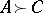relative outcome can change by voters varying theirranking but keeping their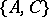rankings fixed. Finally, the procedure has transitive outcomes.

Arrow proved that with more than two alternatives, a dictator is the only procedure satisfying these conditions, i.e., society's ranking is determined by the dictator. Ifis the set of all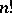transitive rankings of thecandidates, then decision procedures are mappings, where a dictator is an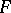that is the identity mapping on one variable; e.g., there is a componentso that for any profile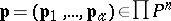, one has.

## Explanation.

With the many extensions (see [a3]) and mathematical proofs of Arrow's theorem, ranging from ultrafilters to geometry [a4] to algebraic topology [a2], it is surprising that it admits an elementary explanation with a benign re-interpretation [a4], [a5]. To explain this, notice that the theorem is meaningless unless voters have transitive preferences. (For instance, if all voters had the same cyclic preferences, then Pareto forces a non-transitive outcome.) However, IIA vitiates this critical transitivity assumption. To see why, for each pair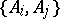, let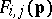be the-relative ranking of; e.g., if, then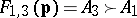. IIA requiresto be decomposed into the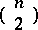mappings, where thedomain depends only on the voters' rankings of. Lettingdenote the set of allways to list the strict rankings of thepairs, IIA requiresto be expressible as. This domain allows IIA-admissible procedures to be used by unsophisticated voters without transitive rankings. Similarly, the implicit independence condition conferred on the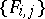functions by IIA renders them incapable of sequencing thepairwise outcomes into transitivity.

Thus, the transitivity assumption is a domain restriction; the goal is to identify those IIA-procedures where the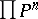restriction is sufficiently severe to force the outcomes to be in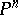. Trivially, this includes a dictator. However, when the rankings of two or more voters are involved, somelevel sets contain transitive and non-transitive profiles, where the non-transitive profile determines a non-transitiveoutcome. Arrow's assertion, then, holds, because IIA prohibits procedures from recognizing transitivity. This permits the alternative interpretation that rational (transitive) outcomes cannot be expected from procedures intended for highly irrational voters.

## Resolution.

To resolve the problem, one replaces IIA with a condition that recognizes rational preferences. One is intensity of irrelevant alternatives (IIIA), where society's relative ranking of two candidates is determined by each voter's relative ranking and the number of alternatives separating them. Replacing Arrow's negative conclusion is that [a4], [a5] the Borda count is a procedure with transitive outcomes satisfying unrestricted domain, IIIA and Pareto. (The Borda count assignspoints to a voter'sth ranked candidate,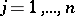; the candidates are ranked according to the sum of assigned points.) Thus, if rational outcomes are desired, use procedures designed for rational voters.

How to Cite This Entry:
Arrow impossibility theorem. Encyclopedia of Mathematics. URL: http://encyclopediaofmath.org/index.php?title=Arrow_impossibility_theorem&oldid=12728
This article was adapted from an original article by D. Saari (originator), which appeared in Encyclopedia of Mathematics - ISBN 1402006098. See original article